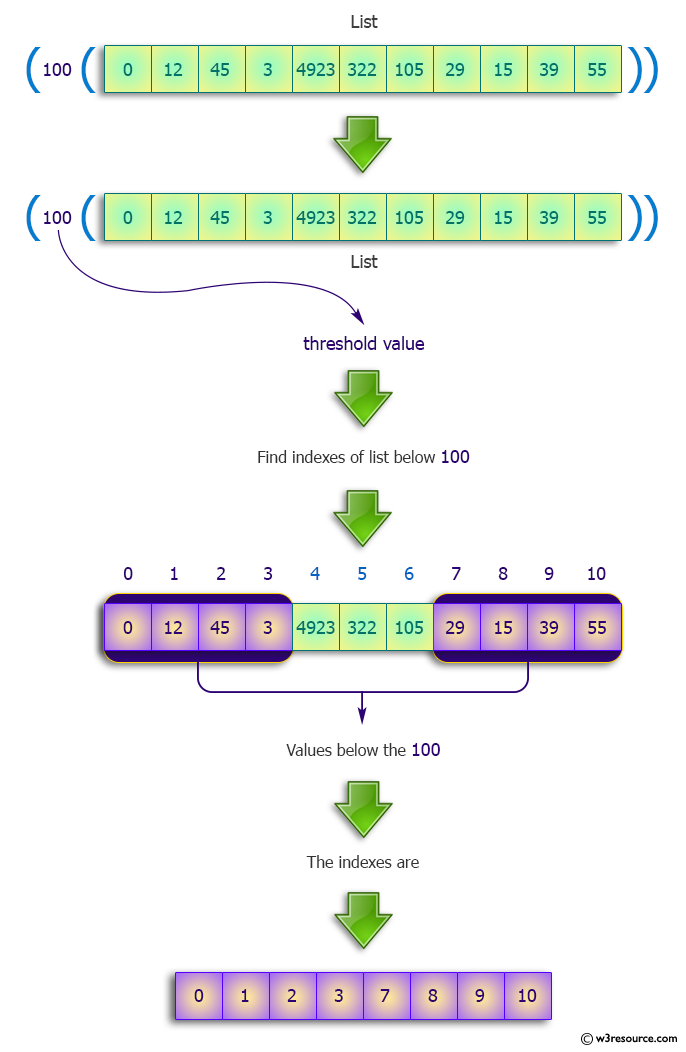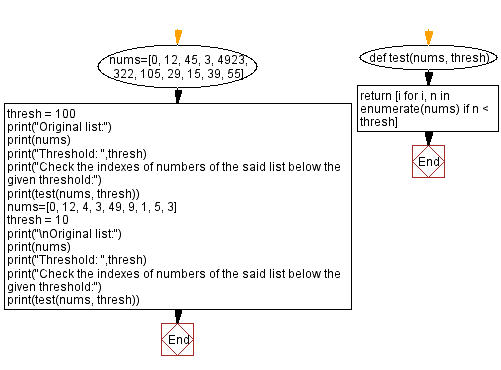﻿ Python: Find the indexes of numbers, below a given threshold - w3resource# Python: Find the indexes of numbers, below a given threshold

## Python Programming Puzzles: Exercise-11 with Solution

Write a Python program to find the indexes of numbers of a given list below a given threshold.

```Input:
[(100,(0, 12, 45, 3, 4923, 322, 105, 29, 15, 39, 55))]
Output:
[0, 1, 2, 3, 7, 8, 9, 10]

Input:
[(10,(0, 12, 4, 3, 49, 9, 1, 5, 3))]
Output:
[0, 2, 3, 5, 6, 7, 8]
```

Pictorial Presentation:Sample Solution:

Python Code:

``````#License: https://bit.ly/3oLErEI

def test(nums, n):
return [i for i,n in enumerate(nums) if n<thresh]

nums=[0, 12, 45, 3, 4923, 322, 105, 29, 15, 39, 55]
thresh = 100
print("Original list:")
print(nums)
print("Threshold: ",thresh)
print("Check the indexes of numbers of the said list below the given threshold:")
print(test(nums, thresh))
nums=[0, 12, 4, 3, 49, 9, 1, 5, 3]
thresh = 10
print("\nOriginal list:")
print(nums)
print("Threshold: ",thresh)
print("Check the indexes of numbers of the said list below the given threshold:")
print(test(nums, thresh))
``````

Sample Output:

```Original list:
[0, 12, 45, 3, 4923, 322, 105, 29, 15, 39, 55]
Threshold:  100
Check the indexes of numbers of the said list below the given threshold:
[0, 1, 2, 3, 7, 8, 9, 10]

Original list:
[0, 12, 4, 3, 49, 9, 1, 5, 3]
Threshold:  10
Check the indexes of numbers of the said list below the given threshold:
[0, 2, 3, 5, 6, 7, 8]
```

Flowchart:## Visualize Python code execution:

The following tool visualize what the computer is doing step-by-step as it executes the said program:

Python Code Editor :

Have another way to solve this solution? Contribute your code (and comments) through Disqus.

What is the difficulty level of this exercise?

Test your Programming skills with w3resource's quiz.

﻿

## Python: Tips of the Day

Clamps num within the inclusive range specified by the boundary values x and y:

Example:

```def tips_clamp_num(num,x,y):
return max(min(num, max(x, y)), min(x, y))
print(tips_clamp_num(2, 4, 6))
print(tips_clamp_num(1, -1, -6))
```

Output:

```4
-1
```